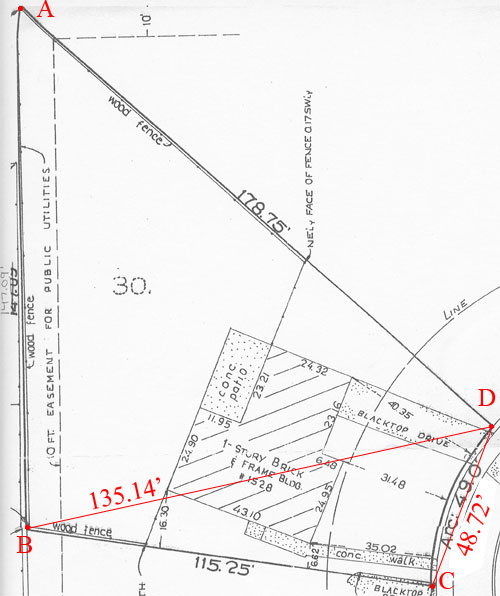SEARCH HOMEMath Central Quandaries & QueriesHi I am attaching a survey of an odd shaped real estate lot. I am hoping you can help me determine the acreage. I was really bad in geometry 20 years ago. Thank you! JaniceHi Janice,Without any angle measurements the best I can do is approximate the area of your lot. I printed the diagram, measured the three side lengths on the printed diagram and then used those measurements to approximate the scale of the drawing. I then used the scale to approximate the lengths from B to D and from C to D as in the diagram. This gives you lot as approximated by two triangles, ABD and BCD. I then used Heron's formula to calculate the areas of the triangles in square feet, added them and convert to acres. My result was 0.2863 acres.

My approximation by triangles ignores the small bite taken out by the arc CD. The diagram gives the arc length as 49.0 feet and the radius of the circle as 66 feet. From this I was able to approximate the area of the bite removed as 0.0033 acres. Hence my approximation of the size of your lot is 0.2863 - 0.0033 acres or approximately 0.28 acres.

HarleyMath Central is supported by the University of Regina and The Pacific Institute for the Mathematical Sciences.